What size have angles in quadrilateral (4-gon) if they are in a ratio of 8: 9: 10: 13?

Result

a =  72 °
b =  81 °
c =  90 °
d =  117 °

#### Solution:Leave us a comment of example and its solution (i.e. if it is still somewhat unclear...):Be the first to comment!#### To solve this example are needed these knowledge from mathematics:

Do you have a linear equation or system of equations and looking for its solution? Or do you have quadratic equation?

## Next similar examples:

1. Supplementary angles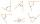One of the supplementary angles are three times larger than the other. What size is larger of supplementary angles?
2. Trapezoid v3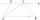The isosceles trapezoid has the angle at a firsst base 40 degrees less than the angle at second base. How large angles has this trapezoid ?
3. 9-gon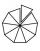Sum of interiol angles of 9-gon is:
4. Find the 9Find the missing angle in the triangle and then name triangle. Angles are: 95, 2x+15, x+3
5. Unknown number 24f we add 20, we get 50% of its triple. What is this unknown number?
6. Fifth of the numberThe fifth of the number is by 24 less than that number. What is the number?
7. Obtuse angleWhich obtuse angle is creating clocks at 17:00?
8. CandyPeter had a sachet of candy. He wanted to share with his friends. If he gave them 30 candies, he would have 62 candies. If he gave them 40 candies, he would miss 8 candies. How many friends did Peter have?
9. 1.5 divided1.5 divided by 1 = w divided by 4
10. Mother and daughter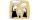The ratio of years mother and daughter is 5:2. After 7 years the ratio is 2: 1. How many years ago daughter was born?
11. Seven tractors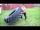Seven tractor plowed 308 ha field in four days. How many hectares of fields will plowe 9 tractors in 3 days at the same power?
12. Boys and girls 2The ratio of boys to girls in math club is 4:3 . After 8 more girls joined the Club, the ratio become 1:1. How many members are there in the club now?Combine like terms 4c+c-7cSolve the equation: 1/2-2/8 = 1/10; Write the result as a decimal number.Identify unknown number which 1/5 is 40 greater than one tenth of that number.Solve for x: 3(x + 2) = x - 18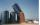The bakery tray for flour was filled to 3/4 volume. After removing 875 kg of flour, it was filled to only 2/5 of the volume. How many tons of flour is in the full tray?